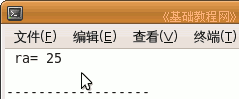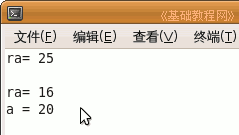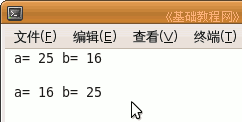C++ 编程基础教程

引用和指针类似，都是直接对变量地址操作，区别是引用对象不能改变，引用要直观好理解一些，下面我们来看一个练习；

1、启动 Geany

1）点菜单“应用程序－编程－Geany”启动 Geany ，新建一个 c++ 源程序；

2）点菜单“文件－另存为”命令，以“refer”为文件名，保存文件到自己的文件夹；

2、建立引用

1）引用相当于变量的别名或另外的绰号，本身并不是变量，因此只有声明没有定义；

输入下面的代码：

 int a = 25;    int &ra = a;    cout << "ra= " << ra;

2）第一句定义一个整型变量 a，变量在内存中有地址，可以用变量名 a 来访问，

第二句建立一个引用，类型是整型，与号 & 是定义符号，前面有类型的一定是声明或定义，

第三句是显示 ra，跟指针类似，定义完了就可以直接用 ra 这个名称；输出 ra 实际上就是输出 a，像是变量 a 又多了一个地址名；

3）接下来我们来看二者之间相互对应关系，继续输入下面的代码；

 a = 16;    cout << "ra= " << ra <

4）第一句改变变量 a 的值，ra 跟着也改变，

第二句改变引用 ra 的值，a 也跟着变；#include using namespace std;int main(int argc, char** argv){    int a = 25;    int &ra = a;    cout << "ra= " << ra;    cout << endl << endl;        a = 16;    cout << "ra= " << ra <

5）引用和指针用在函数中比较多，函数运行时要使用形参，形参是一个局部临时变量，

函数在结束时要返回运算结果，这个值也要先存放在一个局部临时变量里，

使用引用和指针的地址特性，可以跳过局部变量，直接对传过来的变量进行操作，提高速度，节省内存资源；

6）新建一个c++程序，保存文件名为 swap，输入下面的代码；

 #include using namespace std;void swap (int &rx, int &ry); //声明一个函数，定义在主函数外面int main(int argc, char** argv) //主函数，调用 swap 函数{    int a =25;    int b= 16;        cout << " a= " << a;    cout << " b= " << b;        cout << endl << endl;        swap(a,b);  //调用函数，直接交换 a,b        cout << " a= " << a;    cout << " b= " << b;    return 0;}void swap(int &rx, int &ry) //定义前面声明的函数{    int temp = rx;    rx = ry;  //使用的时候直接使用引用名称    ry = temp;}本节学习了引用的基本操作，如果你成功地完成了练习，请继续学习；